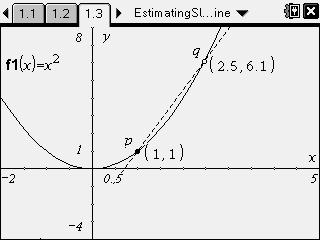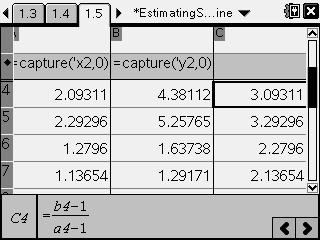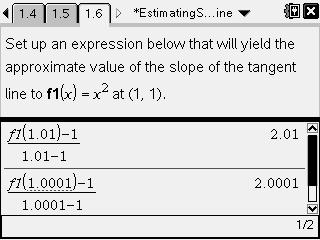# Activities

••• ##### Subject Area

• Math: Calculus: Derivatives

• ##### Author9-12

15 Minutes

• ##### Device
• TI-Nspire™ Navigator™
• TI-Nspire™
• TI-Nspire™ CAS
• ##### Software

TI-Nspire™
TI-Nspire™ CAS

2.0

• ##### Report an Issue

Estimating Slope of a Tangent Line#### Activity Overview

Students examine the graph of y = x2 and secant line pq.

#### Key Steps

•In this activity, students will estimate the slope of a tangent line to a curve by using the secant line between two points on the graph of y = x2.

•First, they will see the effect on the slope of the secant line as the two points are moved closer to each other. They will collect the points in a table of values and calculate the slope for each set of points.

•Finally, they will develop an expression to estimate the slope of the tangent line at the point (1, 1) by using the slope formula.